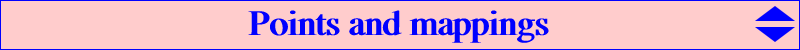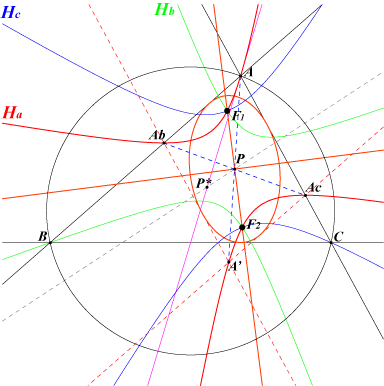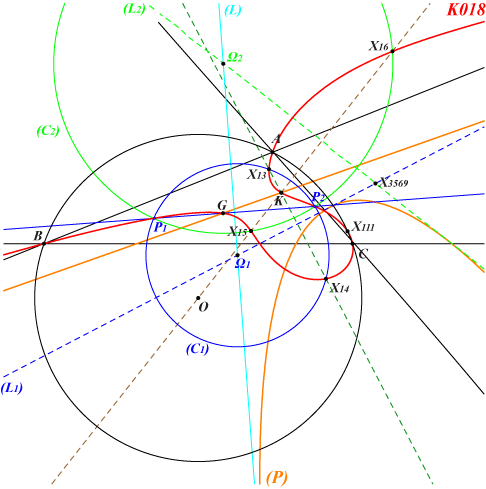antigonal The antigonal N of a point M is equivalently : the isogonal conjugate of the inverse in the circumcircle of the isogonal conjugate of M, the antipode of M on the rectangular circumhyperbola passing through M (hence the midpoint of MN lies on the nine-point circle), the common point of the circles BCA', CAB', ABC' and A'B'C' where A', B', C' are the reflections of M in the sidelines of ABC. the unique point such that (MA,MB)+(NA,NB)=(MB,MC)+(NB,NC)=(MC,MA)+(NC,NA) = 0 (oriented line angles), definition by Jan van Yzeren, 1941. See also Mathematics Magazine, vol. 65, n°. 5, December 1992. The antigonal image of a line is, in general, a bicircular quintic. See K186 for details. See also Q030 and CL019 for antigonal cubics.symgonal The symgonal Q of a point P is equivalently defined as follows : if A', B' ,C' are the reflections of A ,B, C through a point P, the circles BCA', CAB', ABC' have a common point Q which is called the symgonal of P. (Jean-Pierre Ehrmann) the circles AB'C', BC'A', CA'B' have a common point Q' which is also on the circumcircle and the circumconic with center P. Q is the reflection of Q' in P. (Paul Yiu) Q is the antigonal of the anticomplement of P (or P is the complement of the antigonal of Q).GST Consider the mapping f : M(x:y:z) --> M'(x':y':z') given in barycentric coordinates by : x' = x (b^2 SB / y - c^2 SC / z) / a^2 , y' and z' likewise. When M is a point on the circumcircle, M' is the tripole of the Simson line of M and therefore lies on the Simson cubic K010. A geometric construction of GST(M) is the following : the trilinear polar of X(69) meets the sidelines of ABC at U, V, W. N is the isotomic conjugate of the isogonal conjugate of M. Its trilinear polar L meets the sidelines of ABC at A', B', C'. The conic (Ca) through A, V, W, A' tangent at A' to BC meets L at A' and M'. See also the preamble before X(2394) in Clark Kimberling's ETC. GST transforms any line passing through O into an inscribed conic whose perspector is the isotomic conjugate of the isogonal conjugate of the trilinear pole of the line. Since this tripole lies on the circumconic with center X(6), the perspector is a point of the circumconic with perspector X(69). In particular, GST transforms the Brocard axis into the Kiepert parabola.GSC There are always two points M and N such that GST(M) = GST(N) = P. These points are then said to be conjugated under the GSC transformation. In other words, GST(M) = GST(N) <=> N = GSC(M) <=> M = GSC(N). N is in fact the isogonal conjugate of the cevian quotient of H (orthocenter) and M* (isogonal conjugate of M), also N is the X(3)-crossconjugate of M. See exemples of such pairings in the list below. Given a fixed point Q, the locus of M such that M, GSC(M), Q are collinear is a pK passing through Q, O = X(3), with pivot P = Ceva point of Q and O (see glossary in ETC), invariant under the isoconjugation which swaps P and O. For example, with Q = X(155), it is K006 (orthocubic), with Q = X(1993), it is K045 (Euler perspector cubic) and with Q = X(6), it is K168. A simultaneous construction of GSC(M) and GST(M) for M ≠ O is the following. Draw the circumconic (C) passing through M and tangent at M to the line OM. The polar line of O in (C) meets (C) at M and N = GSC(M). The isotomic conjugate of the isogonal conjugate of the perspector of (C) is P = GST(M) = GST(N). Note that GSC transforms the circumcircle of ABC into Q120, the isogonal transform of the nine point circle. More generally, GSC transforms the circum-conic with perspector X into the isogonal transform of the bicevian conic C(H, X*) where X* is the isogonal conjugate of X. Peter Moses contributed a selection of these points M, N, P = X(i), X(j), X(k) for these i, j, k with i < j.i ; j ; k 1 ; 90 ; 4391 2 ; 69 ; 3267 4 ; 254 ; 14618 6 ; 8770 ; 523 21 ; 283 ; 15411 24 ; 14517 ; 15423 25 ; 15369 ; 2489 31 ; 15370 ; 2484 32 ; 15371 ; 669 39 ; 15372 ; 2528 48 ; 15373 ; 1459 54 ; 96 ; 15412 55 ; 15374 ; 4130 56 ; 15375 ; 3669 58 ; 15376 ; 7192 59 ; 100 ; 2397 63 ; 77 ; 15413 71 ; 15377 ; 4064 74 ; 10419 ; 2394 78 ; 271 ; 15416 81 ; 272 ; 15417 95 ; 97 ; 15414 98 ; 2065 ; 2395 99 ; 249 ; 2396 101 ; 15378 ; 2398 102 ; 15379 ; 2399 103 ; 15380 ; 2400 104 ; 15381 ; 2401 105 ; 15382 ; 2402 106 ; 15383 ; 2403 107 ; 15384 ; 2404 108 ; 15385 ; 2405 109 ; 15386 ; 2406 110 ; 250 ; 2407 111 ; 15387 ; 2408 112 ; 15388 ; 2409 184 ; 15389 ; 3049 187 ; 15390 ; 1649 248 ; 15391 ; 879 264 ; 5392 ; 15415 265 ; 15392 ; 14592 284 ; 15393 ; 7253 394 ; 15394 ; 4143 476 ; 15395 ; 2410 477 ; 15396 ; 2411 485 ; 486 ; 850 662 ; 7045 ; 15418 675 ; 15397 ; 2412 895 ; 15398 ; 14977 911 ; 15399 ; 2424 1073 ; 15400 ; 14638 1141 ; 15401 ; 2413 1176 ; 1799 ; 4580 1292 ; 15402 ; 2414 1293 ; 15403 ; 2415 1294 ; 15404 ; 2416 1295 ; 15405 ; 2417 1296 ; 15406 ; 2418 1297 ; 15407 ; 2419 1333 ; 15408 ; 3733 1437 ; 15409 ; 7254 1444 ; 1790 ; 15419 1791 ; 1798 ; 15420 2420 ; 15410 ; 3233 5504 ; 12028 ; 15421 8884 ; 14518 ; 15422 8946 ; 8948 ; 2501In the red lines, one of the points M, N lies on the circumcircle, the other lies on Q120 and then P lies on K010. In the blue lines, one of the points M, N lies on the Brocard axis, the other lies on a quintic and then P lies on the Kiepert parabola.CSTOrion transformations OT, OP, OC See Table 11Psi and its generalization Psi_P The Psi quadratic involution is described here where a list of pairs {P, Psi(P)} is given. Its is a special (and probably the most interesting) case of the transformation Psi_P defined and studied below. Psi is Psi_X2 or Psi_G. See also the paper Cubics passing through the foci of an inscribed conic. Let P = p : q : r be a finite point not lying on the sidelines of ABC. Ha is the rectangular hyperbola with center P, passing through A whose asymptotes are the parallels at P to the A-bisectors of ABC. Hb and Hc are defined cyclically.The equation of Ha is : (p+q+r) (b^2 z^2–c^2 y^2) + 2 (x+y+z) (c^2 q y–b^2 r z) = 0. Ha also passes through : • A' = reflection of A in P, • Ab = AB /\ reflection of AC in P, • Ac = AC /\ reflection of AB in P, • F1, F2 real foci of the inconic with center P and also the imaginary foci F'1, F'2. The tangent at A to Ha passes through the isogonal conjugate P* of P. *** Ha, Hb, Hc are obviously in a same pencil since (∑ a^2 Ha) identically vanishes.(∑ p x Ha) is the equation of pK(X6, P) which confirms that the three hyperbolas pass through the four foci mentioned above. More generally, if Q = u : v : w is another point, (∑ u x Ha) is the equation of spK(Q, P) as in CL055. *** The Psi_P transform Psi_P(M) of a point M is the pole of M in the pencil above. Psi_P can be seen as the isogonal conjugation in the non proper triangle whose vertices are those of the diagonal triangle of the four foci, namely P and the circular points at infinity J1, J2. See Table 62 for other similar transformations. It follows that Psi_P is a quadratic involution with singular points P, J1, J2 and fixed points F1, F2, F'1, F'2. If P is not an in/excenter of ABC, Psi_P(P*) lies on the circumcircle (O) : it is the isogonal conjugate of the infinite point of the line PP*. Hence, the Psi_P image of (O) is a circle C_P passing through P* analogous to the Brocard circle obtained when P = X(2). The center of C_P is the Psi_P image of the inverse of P in (O). C_P also contains the Psi_P images Ap, Bp, Cp of A, B, C which are the vertices of a triangle (analogous to the second Brocard triangle) perspective at P* to ABC. This triangle is equilateral if and only if P = X(15) or X(16). *** A Psi_P pivotal cubic is the locus of M such that M, Psi_P(M) and a fixed point Q are collinear. This cubic is a focal cubic passing through P, J1, J2, F1, F2, F'1, F'2, Q, the infinite point of the line PQ (which is the orthic line) and Psi_P(Q) which is the singular focus F. The polar conic of F is the circle passing through F, P, Q. When Q = P*, this focal cubic is an isogonal nK, locus of foci of inconics with center on the line PP*. *** Psi2 With P = X(6), the transformation Psi_P is Psi2 as mentioned in K018, K1142 and several other pages. Here is a list of pairs {P, Psi2(P)} by Peter Moses (updated 2022-06-05). {1, 5540}, {2, 111}, {3, 115}, {4, 112}, {5, 2079}, {11, 46408}, {13, 16}, {14, 15}, {23, 6032}, {25, 1560}, {32, 6033}, {39, 12188}, {52, 39839}, {54, 13527}, {61, 6777}, {62, 6778}, {64, 13526}, {69, 36696}, {74, 6794}, {98, 34235}, {99, 14700}, {101, 6788}, {110, 6792}, {114, 5941}, {125, 35901}, {128, 15551}, {132, 46942}, {136, 50364}, {182, 11646}, {187, 381}, {265, 32761}, {352, 5640}, {353, 9140}, {378, 5523}, {389, 39849}, {399, 15544}, {403, 21397}, {427, 8428}, {574, 11632}, {599, 28662}, {729, 6787}, {843, 9144}, {868, 8429}, {895, 48945}, {919, 10773}, {1083, 3125}, {1113, 8426}, {1114, 8427}, {1316, 3569}, {1326, 32431}, {1344, 8105}, {1345, 8106}, {1351, 5477}, {1379, 31862}, {1380, 31863}, {1511, 15538}, {1570, 39899}, {1587, 13283}, {1588, 13282}, {1593, 40234}, {1625, 31850}, {1637, 14685}, {1648, 9129}, {1691, 3818}, {1692, 18440}, {1879, 32762}, {1992, 10765}, {1995, 5913}, {2030, 47353}, {2076, 19130}, {2080, 5475}, {2088, 18332}, {2395, 46130}, {2453, 2492}, {2459, 6564}, {2460, 6565}, {2502, 9169}, {2549, 11579}, {2715, 11005}, {3003, 15928}, {3016, 41330}, {3018, 14687}, {3070, 49371}, {3071, 49372}, {3098, 6034}, {3102, 35824}, {3103, 35825}, {3120, 46407}, {3124, 5108}, {3258, 50363}, {3269, 18338}, {3815, 33900}, {5000, 5001}, {5060, 45924}, {5091, 24290}, {5099, 50381}, {5104, 5476}, {5164, 45923}, {5166, 11188}, {5309, 35002}, {5355, 47618}, {5469, 10645}, {5470, 10646}, {5471, 5611}, {5472, 5615}, {5526, 5902}, {5622, 35902}, {5653, 35606}, {5870, 19115}, {5871, 19114}, {5890, 13509}, {5912, 5968}, {5946, 45769}, {5971, 9465}, {6128, 15919}, {6141, 13636}, {6142, 13722}, {6566, 13665}, {6567, 13785}, {6644, 49123}, {6776, 10766}, {6785, 26714}, {7698, 9998}, {7737, 9970}, {7753, 9301}, {9721, 47391}, {10546, 11647}, {10605, 15341}, {10606, 44909}, {10657, 30440}, {10658, 30439}, {11472, 16303}, {11477, 41672}, {11650, 14431}, {13492, 31961}, {13530, 13531}, {13748, 49270}, {13749, 49271}, {14398, 47285}, {14899, 35609}, {15033, 15340}, {15048, 16010}, {16808, 39554}, {16809, 39555}, {18424, 38225}, {18451, 50387}, {19780, 22797}, {19781, 22796}, {20998, 32525}, {21044, 46410}, {28343, 36990}, {30521, 31840}, {31861, 47322}, {32618, 32619}, {34288, 45019}, {35607, 35608}, {35903, 36202}, {35904, 36203}, {35906, 35909}, {35907, 35908}, {38940, 39024}, {39162, 39163}, {39164, 39165}, {41042, 41407}, {41043, 41406}, {45375, 50374}, {45376, 50375}, {45723, 50149}.Psi2 transforms a circle (C1) passing through the Fermat points X(13), X(14), with center Ω1 on the trilinear polar (L1) of X(523), into a circle (C2) passing through the isodynamic points X(15), X(16), with center Ω2 on the trilinear polar (L2) of X(6), the Lemoine axis. (C1) and (C2) meet at P1, P2 on a line passing through G and on K018 since each point is the Psi2-image of the other. In other words, every pair of points on K018, collinear with G, is invariant. The line passing through Ω1 and Ω2 envelopes the parabola (P) with focus X(111) and directrix the line GK. (P) passes through X(i) for i = 1499, 1640, 3288, 5915, 9209, 50565, 50642, 50643, 50644. (P) is tangent to (L1), (L2), the orthic axis, the trilinear polar of X(253), the axes of the Steiner ellipses. Its vertex lies on the lines {111, 1499}, {524, 5914}, {542, 14858}, {1640, 45294}, {5108, 21448}, {5912, 9209}, {5913, 5915}, {9486, 47077}.The dual conic of (P) is the rectangular hyperbola (H) passing through X(i) for i = 2, 20, 69, 76, 99, 3413, 3414, 3663, 6337, 9772, 11057, 20880, 22339, 22340, 32476, 50639, 50640, 50641. Hence, for every point u:v:w on (H), the line u x + v y + w z = 0 is tangent to (P). Its center X(50567) is the barycentric product X(325) x X(524), SEARCH = 4.67050942049146, on the lines {6,620}, {69,74}, {114,325}, {115,141}, {126,1648} and many others. It is the midpoint of X(69), X(99). The barycentric product X(6) x (H) is the Thomson-Jerabek hyperbola. See K002. (H) meets the circumcircle (O) at X(99) and three other points that lie on K169 = pK(X6, X69), and more generally on infinitely many pKs with pole Ω on K177, pivot P on K141, isopivot P* on K174. For given Ω, P = tgcta(Ω) and P* = gaΩ. K141, K168, K169, K174 and pK(X25, X41361), pK(X206, X6), pK(X3162, X4), pK(X41378, X1670), pK(X41379, X1671) are examples of such cubics. *** Some special cases • when (C1) is the Hutson-Parry circle, (C2) is the Parry circle. • when (C1) is the Dao-Moses-Telv circle, (C2) is the Moses-radical circle and the two circles meet at X(5000), X(5001). • when (C1) is the Lester circle, (C2) is the circle with center X(6140), passing through X(i) for i = 115, 128, 399, 1263, 1511, 2079, 10277, 14367. • when (C1) is the Dao-Parry circle, (C2) is the circle with center X(42657), passing through X(i) for i = 3065, 3464, 5540, 6126. *** The following table gives other Psi2-images of points or curves.points or curves Psi2-images A, B, C vertices A4, B4, C4 of the fourth Brocard triangle traces of the trilinear polar of X(523) on the sidelines of ABC vertices A2, B2, C2 of the second Brocard triangle vertices of the circumcevian triangle of K vertices of the orthocentroidal triangle, the projections of G on the altitudes of ABC. See remark 2 below. line BC circle through K, A2, B4, C4. See remark 3 below. Brocard axis Fermat axis trilinear polar of X(523) Brocard circle Euler line circle with center X(2492), passing through X(i) for i = 6, 111, 112, 115, 187, 1560, 2079, etc circumcircle (O) orthocentroidal circle these cubics are globally invariant, see remark 1 belowRemark 1 : the locus of M such that M, Psi2(M) and a given point P ≠ K are collinear is a focal cubic K(P) globally invariant under Psi2. K(P) passes through K, the foci of the orthic inconic, P, its singular focus F = Psi2(P), the infinite point of the line {X6,P} which is the orthic line. Examples : K(X2) = K018, K(X115) = K1276. When P lies on the line at infinity, F = K for every P. The cubics K(P) form a pencil of focal cubics with singular focus K. Remark 2 : these points lie on K005, K295, K397. They are analogous to the vertices A1, B1, C1 of the first Brocard trianglewhich are the Psi-image of the vertices of the circumcevian triangle of G and the projections of K on the perpendicular bisectors of ABC. Their isogonal conjugates lie on K005, K297, K397. They are the vertices of the orthocentroidal-isogonic triangle. Remark 3 : this circle is analogous to the A-McCay circle, the Psi-image of the sideline BC, which passes through G, A4, B2, C2.